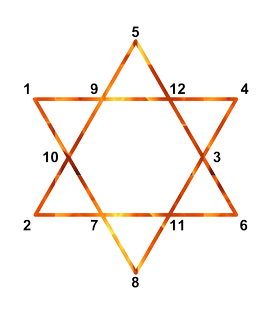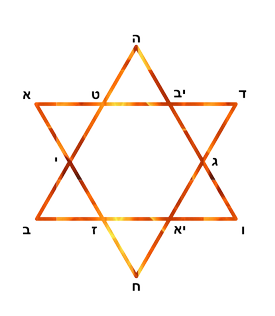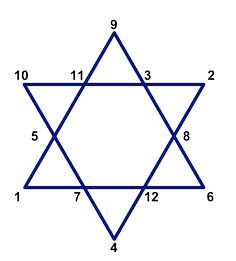## Super Magic Star

Vladimir Goldgefter on the mathematical properties of a six-pointed star
December, 2015

Numbers 1 to 12 can be placed in 12! different ways in a hexagram (Star of David), which is equal to 479 001 600. The arrangement of numbers in the hexagram that I devised is linked to Hebrew letters, gematria, and Kabbalah. It is infused with symmetry, which, according to the great Henri Poincaré, lies at the heart of matter and the universe.

Such a magical hexagram is based on the number 13 and therefore can be a talisman protecting a person as the gematria of the Hebrew word אהבה - "love", or conversely used by occultists to invoke Satan, since in Russian the number 13 is called "a demon's dozen."

May my hexagram bring you love! Here it is, with the usual (left) and Hebrew (right) numbers:Why is this star magic? Recall the magic squares. A magic square is a square table filled with numbers in such a way that the sum of the numbers in each row, each column and on both diagonals is the same.

By analogy with a magic square, consider a magic star. The hexagram consists of two triangles:

• sums of numbers along triangles sides: 1+9+12+4 = 4+3+11+8 = 8+7+10+1 = 5+12+3+6 = 6+11+7+2 = 2+10+9+5 = 26 = 13 x 2
• sums of numbers in triangles vertices: 5+6+2 = 1+4+8 = 13
• large circle: 5+4+6+8+2+1 = 26 = 13 x 2
• small circle: 9+12+3+11+7+10 = 52 = 13 x 4
• cross: vertical line 5+8 = 13 , horizontal line: 10+3 = 13
• large horizontal rectangle: 1+4+6+2 = 13
• smaller horizontal rectangles: 1+12+11+2 = 9+4+6+7 = 13 x 2
• small vertical rectangle: 9+12+11+7 = 39 = 13 x 3
• small vertical triangles: 9+5+12 = 7+11+8 = 26 = 13 x 2
• outer left and right triangles: 1+10+2 = 3+4+6 = 13
• inner left and right triangles: 9+7+10 = 12+3+11 = 26 = 13 x 2

An example of another magic star:• sums of numbers along triangles sides: 10+11+3+2 = 2+8+12+4 = 4+7+5+10 = 9+3+8+6 = 6+12+7+1 = 1+5+11+9 = 26 = 13 x 2
• cross: vertical line 9+4 = 13, horizontal line: 5+8 = 13
• smaller horizontal rectangles: 10+3+12+1 = 11+2+6+7 = 13 x 2
• small triangles on the diagonal: 1+5+7 = 3+2+8 = 13
• small triangles on the other diagonal: 10+11+5 = 8+6+12 = 13 x 2

As one can see, in the first star above the number 13 is contained more times. Therefore I call it a Super Magic Star.

I came up with this star more than 30 years ago, in the mid-1980s. But in those years, in the Soviet Union, publishing about the magical properties of a six-pointed star would be perilous, one could be accused of Zionist propaganda. I came up with the second star recently, at the end of 2014.

One can compose a large number of other six-pointed magic stars. One can find in literature and on the Internet descriptions of various magic stars. But I did not find other authors describing my Super Magic Star.